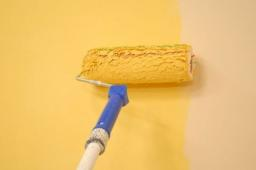# Working together

One painter painted the nursery in 10 days, the second in 8 days. How many days will the nursery be painted if they work together?

x =  4.4444

### Step-by-step explanation:

x·(1/10+1/8)=1

9x = 40

x = 40/9 = 4.44444444

x = 40/9 ≈ 4.444444

Our simple equation calculator calculates it.Did you find an error or inaccuracy? Feel free to write us. Thank you!

Tips for related online calculators
Looking for calculator of harmonic mean?
Looking for a statistical calculator?
Do you have a linear equation or system of equations and looking for its solution? Or do you have a quadratic equation?
Do you want to convert time units like minutes to seconds?